5TH ANNIVERSARY AUCTION

Auction Details

Choose Your Layout >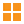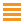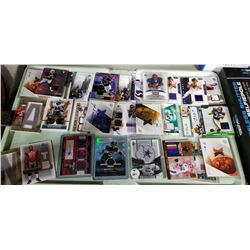101 - Shelf One
CDC Auctions
Shelf One
Bidding Has Concluded

Sold to f***r for (30.00 + 3.90) x 1 = 33.90102 - Shelf One
CDC Auctions
Shelf One
Bidding Has Concluded

Sold to f***r for (80.00 + 10.40) x 1 = 90.40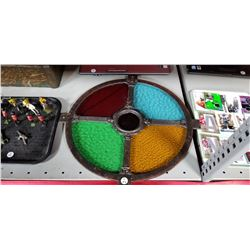103 - Shelf One
CDC Auctions
Shelf One
Bidding Has Concluded

Sold to f***r for (100.00 + 13.00) x 1 = 113.00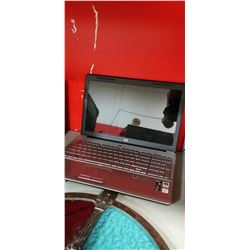104 - Shelf One
CDC Auctions
Shelf One
Bidding Has Concluded

Sold to f***r for (105.00 + 13.65) x 1 = 118.65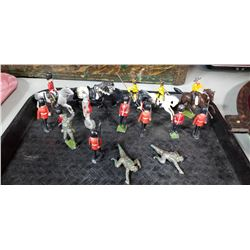105 - Shelf One
CDC Auctions
Shelf One
Bidding Has Concluded

Sold to f***r for (15.00 + 1.95) x 1 = 16.95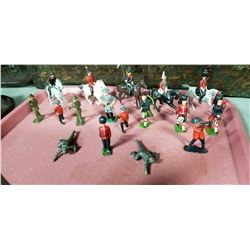106 - Shelf Two
CDC Auctions
Shelf Two
Bidding Has Concluded

Sold to f***r for (25.00 + 3.25) x 1 = 28.25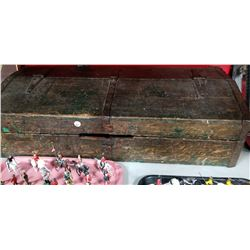107 - Shelf One
CDC Auctions
Shelf One
Bidding Has Concluded

Sold to f***r for (22.50 + 2.93) x 1 = 25.43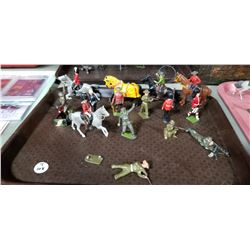108 - Shelf One
CDC Auctions
Shelf One
Bidding Has Concluded

Sold to f***r for (40.00 + 5.20) x 1 = 45.20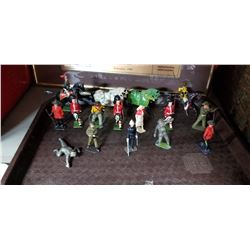109 - Shelf One
CDC Auctions
Shelf One
Bidding Has Concluded

Sold to f***r for (20.00 + 2.60) x 1 = 22.60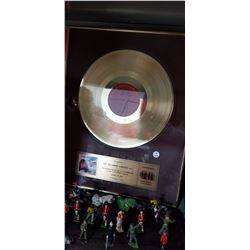110 - Shelf One
CDC Auctions
Shelf One
Bidding Has Concluded

Sold to f***r for (90.00 + 11.70) x 1 = 101.70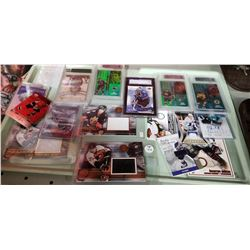111 - Shelf One
CDC Auctions
Shelf One
Bidding Has Concluded

Sold to f***r for (50.00 + 6.50) x 1 = 56.50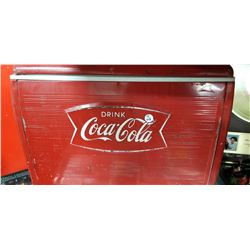112 - Shelf One
CDC Auctions
Shelf One
Bidding Has Concluded

Sold to f***r for (50.00 + 6.50) x 1 = 56.50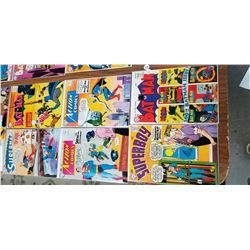113 - Shelf One
CDC Auctions
Shelf One
Bidding Has Concluded

Sold to f***r for (220.00 + 28.60) x 1 = 248.60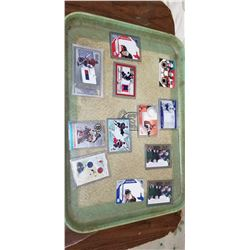114 - Shelf One
CDC Auctions
Shelf One
Bidding Has Concluded

Sold to f***r for (40.00 + 5.20) x 1 = 45.20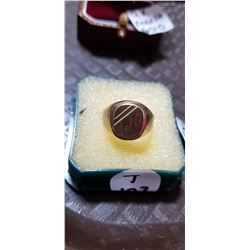115 - Shelf One
CDC Auctions
Shelf One
Bidding Has Concluded

Sold to f***r for (55.00 + 7.15) x 1 = 62.15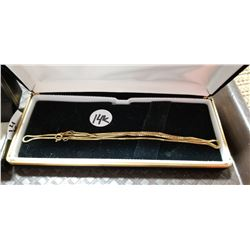116 - Shelf One
CDC Auctions
Shelf One
Bidding Has Concluded

Sold to f***r for (140.00 + 18.20) x 1 = 158.20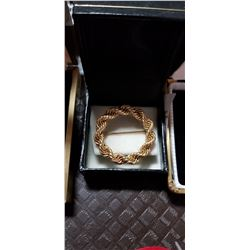117 - Shelf One
CDC Auctions
Shelf One
Bidding Has Concluded

Sold to f***r for (110.00 + 14.30) x 1 = 124.30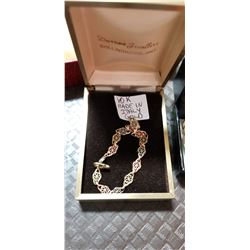118 - Shelf One
CDC Auctions
Shelf One
Bidding Has Concluded

Sold to f***r for (55.00 + 7.15) x 1 = 62.15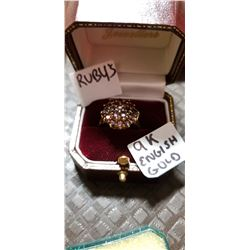119 - Shelf One
CDC Auctions
Shelf One
Bidding Has Concluded

Sold to f***r for (95.00 + 12.35) x 1 = 107.35